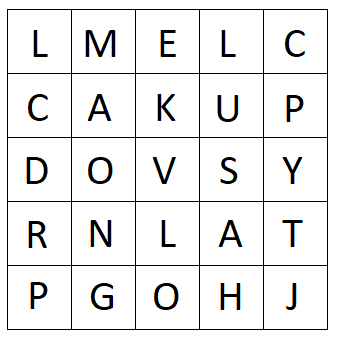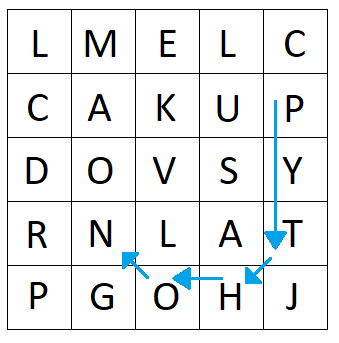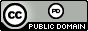시간 제한 메모리 제한 제출 정답 맞은 사람 정답 비율
6 초 1024 MB 0 0 0 0.000%

## 문제

You're probably familiar with regular word searches, where you're presented with a grid of letters and a word to find.  The word can be in a straight line horizontally, vertically, or diagonally (and perhaps backwards in any of those directions).  For example, here is a grid of letters:Figure 1: A word search grid

The word "JAVA" can be found going from the bottom right corner diagonally upwards.

In a kinky word search the path that spells out the word can have one or more "kinks" -- places where the path changes direction.  For example, in the given grid you can spell the word "PYTHON" with $3$ kinks (one each at the T, H and  O):Figure 2: A kinky spelling of "PYTHON"

Adding kinks allows letters to be reused -- the word "CPLUSPLUS" can be found in the upper right corner of the grid (with $5$ kinks).  However you cannot stay on a letter twice in a row, so you cannot spell the word "HASKELL" in this grid (though you can find at least $11$ more common programming languages). Your task is to see if the spelling of a word with a certain number of kinks is possible or not.

## 입력

Input begins with a line containing two positive integers $r$ and $c$ ($r, c \leq 10)$, the number of rows and columns in the grid.  After this are $r$ rows of $c$ uppercase characters.  Letters are separated by a space. After the grid are two lines: The first line is an integer $k$, the number of kinks.  The second line contains an uppercase word to look for, with maximum length $100$.

## 출력

Output either the word YES if it is possible to spell the given word with exactly $k$ kinks on the grid provided, or NO if it is not.

## 예제 입력 1

5 5
L M E L C
C A K U P
D O V S Y
R N L A T
P G O H J
0
JAVA


## 예제 출력 1

YES


## 예제 입력 2

5 5
L M E L C
C A K U P
D O V S Y
R N L A T
P G O H J
3
PYTHON


## 예제 출력 2

YES


## 예제 입력 3

5 5
L M E L C
C A K U P
D O V S Y
R N L A T
P G O H J
4
PYTHON


## 예제 출력 3

NO


## 출처• 문제를 만든 사람: John Bonomo